T or F
Comparing fractions
T or F
Equivalent Fractions
Comparing
Fractions
Equivalent
Fractions
*Challenge*
100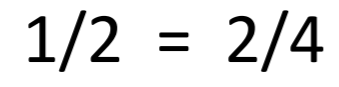True

100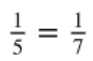false

100

What is the correct symbol?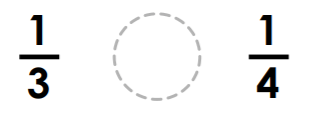>

100

What is the missing numerator?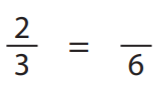4

100

Write the numbers from least to greatest: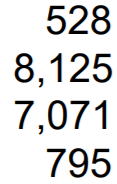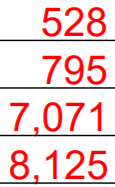200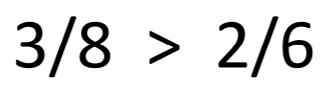True

200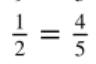False

200

What is the correct symbol?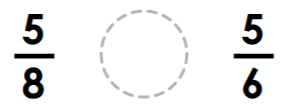<

200

Choose the letter that shows the equivalent fractions: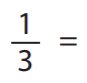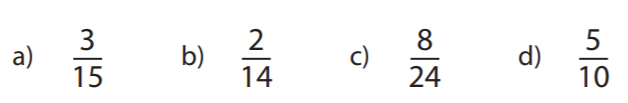C

200

Write the numbers in order from least to greatest: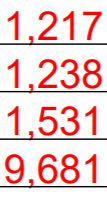300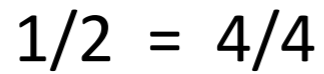False

300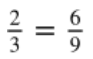True

300

What is the correct symbol?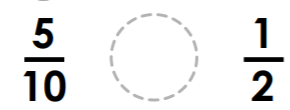=

300

Which letter shows the equivalent fraction?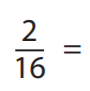B

300

Order the fractions from least to greatest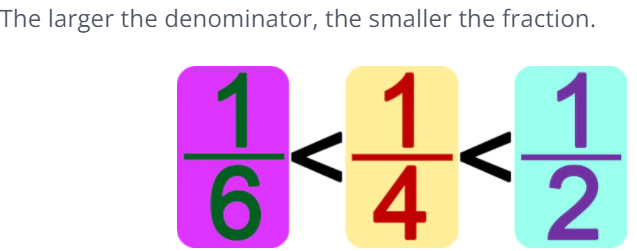400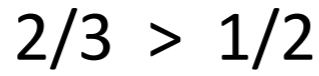True

400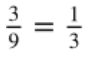True

400

What is the correct symbol?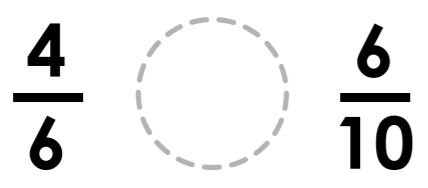>

400

Two parts.

1. What should you multiply by?

2. What is the new numerator?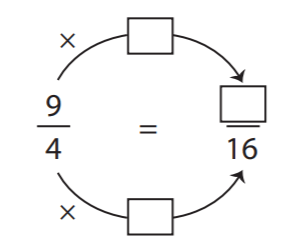Multiply by 4.

Numerator is 36.

400

Order the fractions from least to greatest: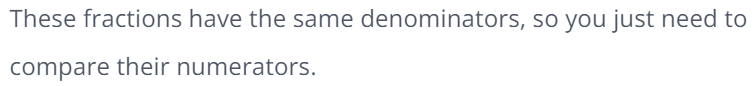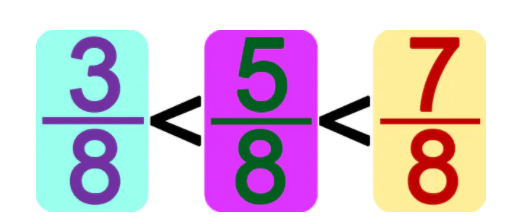500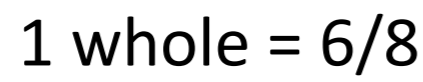False

500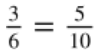True

500

What is the correct symbol?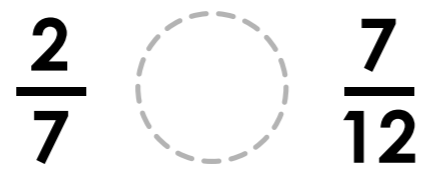<

500

What is the missing denominator?9

500

Order these fractions from least to greatest: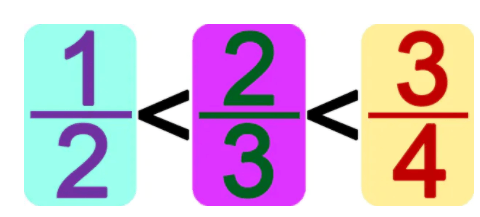Click to zoom